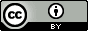## Thursday, January 2, 2014

### EFMO-PCM: A road map

One of the things still missing in the EFMO method is an interface to PCM.  Here I attempt to sketch the method, based on the FMO-PCM and EFP-PCM interfaces.

The PCM electrostatic interaction free energy between solute and solvent is

$G_s=\frac{1}{2}\mathbf{V}^T \mathbf{q}$

$\mathbf{q}$ are the apparent surface charges (ASCs), which for large systems are obtained by solving this equation iteratively

$\mathbf{Cq=-V}$

For EFMO $\mathbf{V}$ is the electrostatic potential from the static multipoles and induced dipoles

$\mathbf{V=V^{\text{mul}}+V^{\mu}}$

The simplest approximation to $\mathbf{V}$ is
\begin{align*} \mathbf{V}& =\sum_I^N \mathbf{V}_{I}\\ & = \sum_I^N (\mathbf{V}^{\text{mul}}_{I}+\mathbf{V}^{\mu}_{I}) \end{align*}
The potential at tesserae $j$ due to induced dipoles on fragment $I$ is given by:
$$\mathbf{V}_I^\mu (j)=\sum_{i \in I} (\mathbf{R}^T)_{ji}\boldsymbol{\mu_{i}}$$
The induced dipoles are obtained iteratively:
$$\boldsymbol{\mu}_i=\boldsymbol{\alpha}_i\left(\mathbf{F}^{\text{mul}}_i+\mathbf{F}^q_i-\sum_{i\neq j}\mathbf{D}_{ij}\boldsymbol{\mu}_j\right)$$
where $\boldsymbol{\alpha}_i$ is the dipole polarizability tensor at site $i$ and $\mathbf{F}^{\text{mul}}_i$ and $\mathbf{F}^q_i$ are the electrostatic fields due to all static multipoles and ASCs felt at point $i$.

Procedure:

1. Compute EFMO gas phase energy

2. Use gas phase static multipoles and $\boldsymbol{\mu}$ to construct $\mathbf{V}$

3. Solve $\mathbf{Cq=-V}$

4. Use $\mathbf{q}$ and $\boldsymbol{\mu}_i=\boldsymbol{\alpha}_i\left(\mathbf{F}^{\text{mul}}_i+\mathbf{F}^q_i-\sum_{i\neq j}\mathbf{D}_{ij}\boldsymbol{\mu}_j\right)$ to find new $\boldsymbol{\mu}$

5. Repeat steps 2-4 until self consistency

6. Compute $G_s$

For more accurate results one  can approximate $\mathbf{V}$ as
$$\mathbf{V}=\sum_I^N \mathbf{V}_{I}+\sum^N_{I}\sum^N_{J<I} (\mathbf{V}_{IJ}-\mathbf{V}_{I}-\mathbf{V}_{J})$$
This essentially means that the gas phase static multipoles and $\alpha$'s are corrected in step 2. E.g. for static monopoles ($q$)'s:
$$V_I^q(i)=\frac{q_i^{I}}{|r-r_i|}$$
and
\begin{align*} V(i)&=V_I^q(i)+\sum^N_{J<I} (V_{IJ}^q(i)-V_I^q(i))\\ & = [q^{I}_i+\sum^N_{J<I}(q^{IJ}_i-q^{I}_i)]\frac{1}{|r-r_i|} \\ \end{align*}
All other steps are the same.This work is licensed under a Creative Commons Attribution 4.0